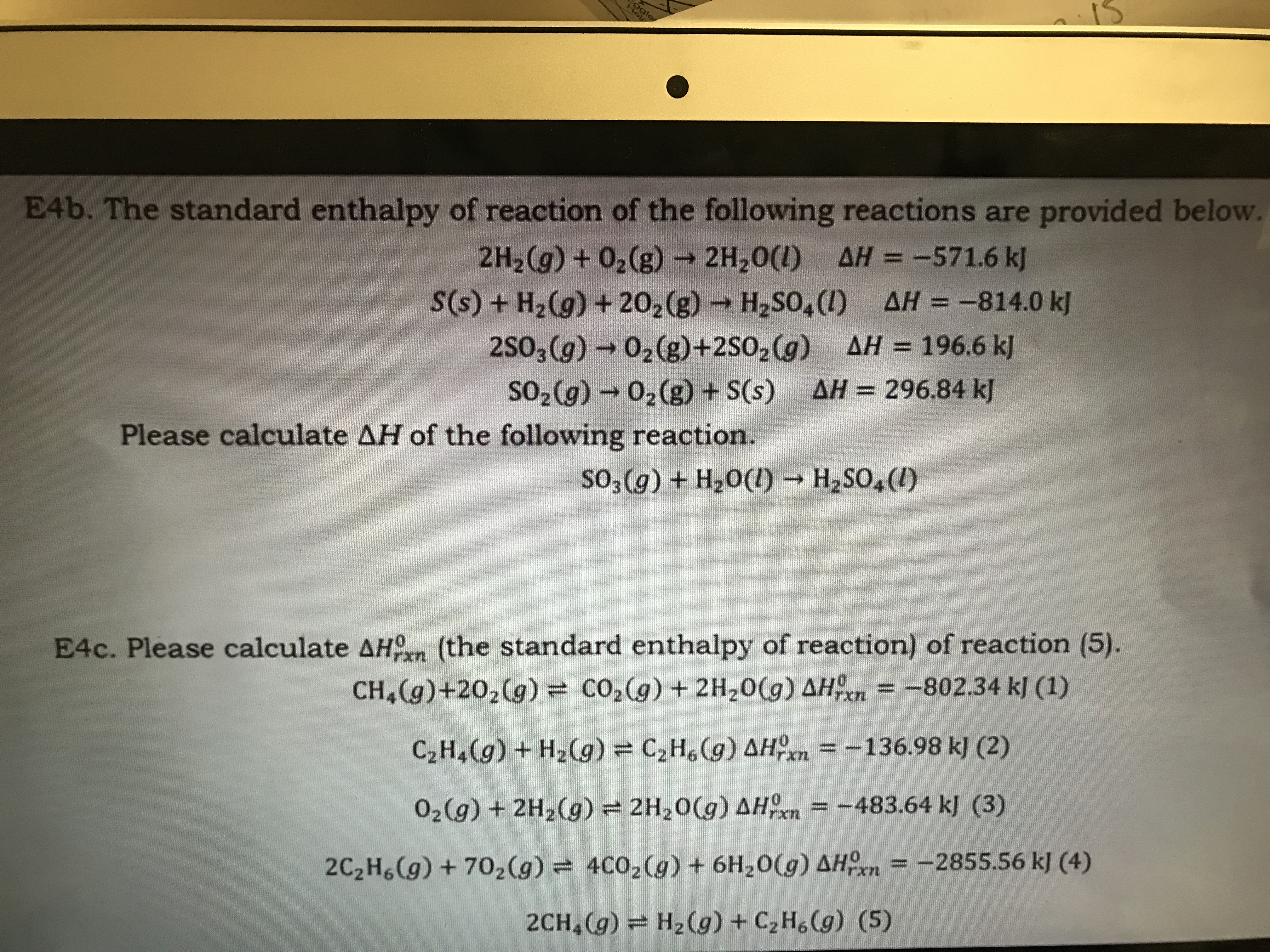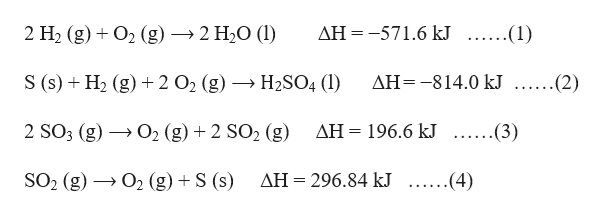# HolE4b. The standard enthalpy of reaction of the following reactions are provided below.2H2(g) +02(g)2H20(I) AH = -571.6 kjS(s)+ H2(g) +202(g) H2SO4() AH-814.0 k]2SO3(g) 02(g)+2SO2 (g) AH 196.6 kSO2(g) 02(g) +S(s) AH = 296.84 kJPlease calculate AH of the following reaction.so3 (g) + H20()H2SO,(I)E4c. Please calculate AH (the standard enthalpy of reaction) of reaction (5).CH&(g)+202(g) = CO2(g) + 2H20(g) AHn -802.34 kJ (1)=-136.98 k] (2)C2H4(g)+H2(g) = C2H6(g) AHOn=-483.64 kl (3)O2(g) + 2H2(g) = 2H20(g) AH4C02(g) + 6H20(g) AHOn = -2855.56 kJ (4)2C2H6(g) +702(g)H2(g) +C2H6(g) (5)2CH (g)

Question
7 views

How do you do E4b?help_outlineImage TranscriptioncloseHol E4b. The standard enthalpy of reaction of the following reactions are provided below. 2H2(g) +02(g)2H20(I) AH = -571.6 kj S(s)+ H2(g) +202(g) H2SO4() AH-814.0 k] 2SO3(g) 02(g)+2SO2 (g) AH 196.6 k SO2(g) 02(g) +S(s) AH = 296.84 kJ Please calculate AH of the following reaction. so3 (g) + H20() H2SO,(I) E4c. Please calculate AH (the standard enthalpy of reaction) of reaction (5). CH&(g)+202(g) = CO2(g) + 2H20(g) AHn -802.34 kJ (1) =-136.98 k] (2) C2H4(g)+H2(g) = C2H6(g) AHOn =-483.64 kl (3) O2(g) + 2H2(g) = 2H20(g) AH 4C02(g) + 6H20(g) AHOn = -2855.56 kJ (4) 2C2H6(g) +702(g) H2(g) +C2H6(g) (5) 2CH (g) fullscreen
check_circle

star
star
star
star
star
1 Rating
Step 1

Given reactions are :help_outlineImage Transcriptionclose2 H2 (g)O2 (g)»2 H20 (l AH -571.6 kJ ......(1) S (s) H2 (g)2 O2 (g)H2SO4 (l) AH814.0kJ ......(2) 2 SO3 (g) AH 196.6 kJ ......(3) O2 (g)2 SO2 (g) SO2 (g)O2 (g)S (s) AH 296.84 kJ .....(4) fullscreen
Step 2

Reversing equation (1) and dividing by 2, the enthalpy value will change in sign and get divided by 2, we get,

Step 3

Dividing equation (3) by 2, so the enthalpy...

### Want to see the full answer?

See Solution

#### Want to see this answer and more?

Solutions are written by subject experts who are available 24/7. Questions are typically answered within 1 hour.*

See Solution
*Response times may vary by subject and question.
Tagged in

### Chemical Thermodynamics